### Home > MC2 > Chapter 2 > Lesson 2.1.2 > Problem2-30

2-30.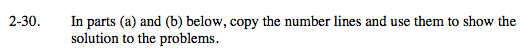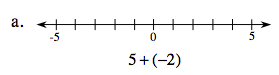The answer is where the point moves to.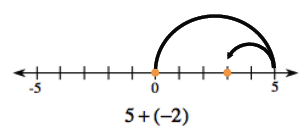3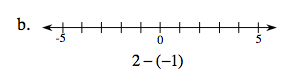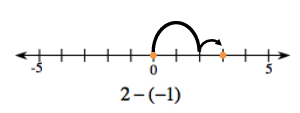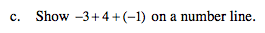See parts (a) and (b) for help.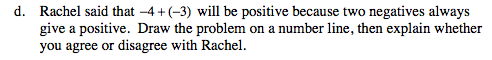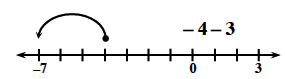Explain why a negative number plus a negative number is always a negative number.

−1 + (−2) = -3
−7 + (−4) = −11